makfile专题

1 篇文章 0 订阅

CSDN 仅用增加百度收录，这里的文章内容不再更新维护。请访问：baron-z.cn 查看、评论，-------------目前网站正在备案可能暂时无法访问，过段时间就能正常访问。

一、make 和 makefile

1、make 的本质

make 是一个应用程序，用于解析源程序之间的依赖关系，根据依赖关系自动维护编译工作，执行宿主操作系统中的各种命令

2、makefile 的本质

makefile 是一个描述文件, 可以 定义一系列的规则来指定源文件编译的先后顺, 拥有特定的语法规则，支持函数定义和函数调用, 能够直接集成操作系统中的各种命令

3、make 和 makefile之间的关系

makefile 中的描述用于指导 make 如何完成工作，make 根据 makefile 中的规则执行命令，最后完成编译输出

4、最简单的 makefle 程序

hello :
@echo “hello makefile”

hello --> 目标
@echo “hello makefile” -> 实现目标所需要执行的命令

make –f mf.txt hello

二、初识 makefile 的结构

1、makefile 的意义

makefile 用于定义源文件之间的依赖关系，说明如何编译各个源文件并生成可执行文件，依赖的定义如下

targets : prerequisite ; command1
‘\t’ command2 #使用这个方式可以省略分号

targets(目标)：
通常是需要生成的目标文件名，make 所需执行的命令名称，可以包含多个目标，使用空格对将多个目标隔开

prerequisite(依赖)：
当前目标所依赖的其他目标文件，可以包含多个依赖，使用空格对将多个依赖隔开，如果省略不写，就意味着只要执行后续的命令那么目标就可以完成了。

command(命令)：
完成目标所需要执行的命令，规则中的注意事项，[tab]键：’\t’ ，每一个命令必须以[tab]字符开始，[tab]字符告诉 make 此行是一个命令行。只要命令成功执行完，则认为目标完成

可以将内容分开写到下一行，提高可读性

3、规则all : test
@echo "make all"

test :
@echo "make test"

make test
make all

3、第一个 make 的编译案例

func.c 文件内容如下

int i = 5;

main.c 文件内容如下

#include <stdio.h>

extern int i;

int main()
{
printf("i = %d\n", i);
return 0;
}

makefile 文件内容如下

hello.out : main.o func.o
gcc -o hello.out main.o func.o
main.o :
gcc -o main.o -c main.c
func.o :
gcc -o func.o -c func.c

gcc -o main.o -c main.c
gcc -o func.o -c func.c
gcc -o hello.out main.o func.o

hello.out all : main.o func.o
gcc -o hello.out main.o fun.o

三、伪目标

1、伪目标的引入

默认情况下，make 认为目标对应着一个文件，make 比较目标文件和依赖文件的新旧关系，决定是否执行命令，make 以文件处理作为第一优先级。但有时候我们希望我们的目录并不是一个文件如下所示：

clean :
rm main.o func.o

这个时候通过 .PHONY 关键字声明一个伪目标, 伪目标不对应任何实际的文件,不管目标的依赖是否更新，命令总是执行, 伪目标的语法如下

.PHONY : Target

.PHONY : clean rebuild all

## other rules ##

rebuile : clean all

clean :
rm *o hello.out

2、绕开 .PHONY 关键字定义伪目标

如果一个规则没有命令或者依赖，并且它的目标不是一个存在的文件名；在执行此规则时，目标总是被认为是最新的。

clean : FORCE
rm *.o hello.out
FORCE :

四、变量和不同的赋值方式

1、makefile中的变量

makefile 中支持程序设计语言中变量的概念，makefile 中变量只代表文本数据。makefile 中变量命名规则如下所示

1. 变量名大小写敏感
2. 变量名可以包含字符，数字，下划线
3. 不能包含 “:” , “#” , “=” , 或 " "
4. makefile 未赋值的变量的值为空值

2、变量的定义和使用CC := gcc
TARGET := hello.out

$(TARGET) : main.o func.o$(CC) -o $(TARGET) main.o func.o main.o : main.c$(CC) -o main.o -c main.c
func.o : func.c
$(CC) -o func.o -c func.c .PHONY : rebuild clean all rebuile : clean all all :$(TARGET)

clean :
rm *.o $(TARGET) 运行结果： gcc -o main.o -c main.c gcc -o func.o -c func.c gcc -o hello.out main.o func.o 3、makefile中变量的赋值方式 1) 简单赋值 := 程序设计语言中的通用赋值方式, 只针对当前语句变量有效 x := foo y :=$(x)b
x := new

.PHONY : test
test :
@echo “x = $(x)” @echo “y =$(y)”

x = new
y = foob

2) 递归赋值 =

x = foo
y = $(x)b x = new .PHONY : test test : @echo “x =$(x)”
@echo “y = $(y)” 输出结果： x = new y = newb 3) 条件赋值 ?= 如果变量未定义，使用赋值符号中的值定义变量, 如果变量已经定义，赋值无效 x := foo y :=$(x)b
x ?= new

.PHONY : test
test :
@echo “x = $(x)” @echo “y =$(y)”

x = foo
y = foob

4) 追加赋值 +=

x := foo
y := $(x)b x +=$(y)

.PHONY : test
test :
@echo “x = $(x)” @echo “y =$(y)”

x = foo foob
y = foob

五、预定义变量的使用

1. 自动变量

@ ∗ ∗ 当 前 规 则 中 触 发 命 令 被 执 行 的 目 标 , 即 当 前 规 则 中 的 目 标 ∗ ∗ @** 当前规则中触发命令被执行的目标, 即当前规则中的目标 ** ^ 当前规则中的所有依赖
$< 当前规则中的第一个依赖 2、自动变量的使用 PHONY : all first second third all : first second third @echo “\$$@ => @” @echo “$$^ => $$^” @echo “$$< =>$<”

first :
second :
third :

$@ => all$^ => first second third
$< => first 小贴士： “$” 对于 makefile 有特殊含义，输出时加上一个 $进行转义 “$@” 对于Bash Shell 有特殊含义，输出时加上 \ 进行转义

CC := gcc
TARGET := hello.out

$(TARGET) : main.o func.o$(CC) -o $@$^
main.o : main.c
$(CC) -o$@ -c $^ func.o : func.c$(CC) -o $@ -c$^

.PHONY : rebuild clean all

rebuile : clean all

all : $(TARGET) clean : rm *.o$(TARGET)

gcc -o main.o -c main.c
gcc -o func.o -c func.c
gcc -o hello.out main.o func.o

3、特殊变量

• $(MAKE): 当前 make 解释器的文件名 •$(MAKECMDGOALS): 命令中指定的目标名（ make 的命令行参数）

• $(MAKEFILE_LIST): make 所需要处理的 makefile 文件列表, 当前 makefile 的文件名总是位于列表的最后, 文件名之间以空格进行划分 •$(MAKE_VERSION): 当前 make 解释器的版本

• $(CURDIR): 当前 make 解释器的工作目录 •$(.VARIABLES): 所有已经定义的变量名列表，其中包括预定义变量和自定义变量

.PHONY : all out test first second third

all out : first second third
@echo "$(MAKE)" @echo "$(MAKECMDGOALS)"
@echo "$(MAKEFILE_LIST)" first : @echo "first" second : @echo "second" third : @echo "third" test : @$(MAKE) first
@$(MAKE) second @$(MAKE) third

first
second
third
make

makefile

make: Entering directory '/home/book/c/mkfile'
first
make: Leaving directory '/home/book/c/mkfile'
make: Entering directory '/home/book/c/mkfile'
second
make: Leaving directory '/home/book/c/mkfile'
make: Entering directory '/home/book/c/mkfile'
third
make: Leaving directory '/home/book/c/mkfile'

.PHONY : test1 test2

test1 :
@echo "$(MAKE_VERSION)" @echo "$(CURDIR)"
@echo "$(.VARIABLES)" 运行结果： 4.1 /home/book/c/mkfile <D ?F .SHELLFLAGS CWEAVE ?D @D @F MAKE_VERSION CURDIR SHELL RM CO COMPILE.mod _ PREPROCESS.F LINK.m LINK.o OUTPUT_OPTION COMPILE.cpp MAKEFILE_LIST GNUMAKEFLAGS LINK.p XDG_DATA_DIRS DBUS_SESSION_BUS_ADDRESS CC CHECKOUT,v LESSOPEN CPP LINK.cc SSH_CONNECTION PATH LD TEXI2DVI YACC SSH_TTY XDG_RUNTIME_DIR ARFLAGS LINK.r LINT COMPILE.f LINT.c YACC.m YACC.y AR .FEATURES TANGLE LS_COLORS GET %F DISPLAY COMPILE.F CTANGLE .LIBPATTERNS LINK.C PWD LINK.S PREPROCESS.r *D LINK.c LINK.s HOME LESSCLOSE LOGNAME ^D MAKELEVEL COMPILE.m MAKE SHLVL AS PREPROCESS.S COMPILE.p XDG_SESSION_ID USER FC .DEFAULT_GOAL %D WEAVE MAKE_COMMAND LINK.cpp F77 OLDPWD .VARIABLES PC *F COMPILE.def LEX ARCH MAKEFLAGS MFLAGS SSH_CLIENT MAIL LEX.l LEX.m +D COMPILE.r MAKE_TERMOUT +F M2C CROSS_COMPILE MAKEFILES COMPILE.cc <F CXX COFLAGS COMPILE.C ^F COMPILE.S LINK.F SUFFIXES COMPILE.c COMPILE.s .INCLUDE_DIRS .RECIPEPREFIX MAKEINFO MAKE_TERMERR OBJC MAKE_HOST TEX LANG TERM F77FLAGS LINK.f 六、变量的高级主题 1. 常用语法 1） 变量值的替换 使用指定字符（串）替换变量中的后缀字符（串），语法格式如下$(var:a=b) 或 ${var:a=b} 替换表达式中不能有任何的空格，make 中支持使用${} 对变量进行取值

src := a.cc b.cc c.cc
obj := $(src:cc=o) test : @echo ”obj =>$(obj)”

2）变量的模式替换

$(var:a%b=x%y)或${var:a%b=x%y}

.PHONY : test

test :
@echo "obj => $(obj)" 输出结果： obj => x1y x2y x3y 3）规则中的模式替换 targets : targets-pattern : prereq-pattern command1 command2 … 当 targets(目标) 的依赖的规则不存在时，通过 targets-pattern 从 targets 中匹配子目标,再通过 prereq-pattern 从子目标生成依赖, 进而构成完整的规则, 举例说明如下。 .PHONY : rebuild clean all CC := gcc TARGET := hello.out OBJS := main.o func.o$(TARGET) : $(OBJS)$(CC) -o $@$^

##############################
$(OBJS) : %.o : %.c // 规则中的模式替换$(CC) -o $@ -c$^
#############################

rebuild : clean all

all : $(TARGET) clean : rm -rf *.o$(TARGET)

.PHONY : rebuild clean all

CC := gcc
TARGET := hello.out
OBJS := main.o func.o

$(TARGET) :$(OBJS)
$(CC) -o$@ $^ ############################## mian.o : main.c gcc -o mian.o -c mian.c func.o : func.c gcc -o func.o -c func.o ############################# rebuild : clean all all :$(TARGET)

clean :
rm -rf *.o $(TARGET) 除此之外呢，我们还可以省略掉 targets 如下所示 .PHONY : rebuild clean all CC := gcc TARGET := hello.out OBJS := main.o func.o$(TARGET) : $(OBJS)$(CC) -o $@$^

##############################
%.o : %.c   // 规则中的模式替换
$(CC) -o$@ -c $^ ############################# rebuild : clean all all :$(TARGET)

clean :
rm -rf *.o $(TARGET) 示例代码在大部分情况可以理解为等价的，特殊情况如下 %.o : %.c$(CC) -o $@ -c$^

$(OBJS) : %.o : %.c // 规则中的模式替换$(CC) -o $@ -c$^

4）变量值的嵌套引用

x := y
y := z
a := $($(x))

test :

6）override 关键字

override var := test
test :
$(cmd) 运行结果： I’m fool: run cmd begin ... run cmd end ... 2、环境变量（全局变量） makefile 中能够直接使用环境变量的值，定义了同名变量，默认环境变量将被覆盖。运行 make 时指定 “-e” 选项使用系统默认环境变量。环境变量可以在所有的 makefile 中使用，过多的依赖环境变量会使系统的移植性降低。 变量在不同的 makefile 中的传递方式有三种：直接在外部定义环境变量进行传递、使用 export 定义变量进行传递(定义临时环境变量)、定义 make 命令行变量进行传递(推荐)。 编程实验, makefile 文件如下 PWD := pwd # 修改系统环境变量 export var := D.T.Software # 定义临时环境变量 version := v1 # 普通变量 user :=baron # 普通变量 test : @echo "PWD ==>$(PWD)"
@echo "make another file ..."
$(MAKE) -f makefile2$(MAKE) -f makefile2 user:=$(user) # 将 user 这个变量通过 make 进行传递 makefile2 文件如下 test : @echo "PWD ==>$(PWD)"
@echo "var = $(var)" @echo "version =$(version)"
@echo "user = $(user)" 运行结果如下 PWD ==> pwd make another file ... make -f makefile2 make: Entering directory '/home/book/c/mkfile' PWD ==> pwd var = D.T.Software version = user = make: Leaving directory '/home/book/c/mkfile' make -f makefile2 user:=baron make: Entering directory '/home/book/c/mkfile' PWD ==> pwd var = D.T.Software version = user = baron make: Leaving directory '/home/book/c/mkfile' 3、目标变量（局部变量） 作用域只在指定目标连带规则中 语法格式 target : variable_name := variable-value 举例说明 var := D.T.Software test : var := test-var test : test1 @echo "test:" @echo "var =>$(var)"

test1 :
@echo "test1:"
@echo "var ==> $(var)" test2 : @echo "test2:" @echo "var ==>$(var)"

book@100ask:~/c/mkfile$make test1 var ==> test-var test: var => test-var book@100ask:~/c/mkfile$

rule:
new => test-new

七、条件判断语句

1、makefile中的条件判断语句

ifxxx (arg1,arg2) #注意括号里面不能使用空格
# for true
else
# for false
endif

ifxxx "arg1" "arg2"
ifxxx "arg1" 'arg2'
ifxxx 'arg1' "arg2"
ifxxx 'arg1' "arg2'

2、条件判断关键字

ifeq ( ( x ) , (x), (y))判断参数是否相等，相等为 true ，否则为 false
ifneq ( ( x ) , (x), (y))判断参数是否不相等，不相等则为 true，否则为false
ifdef x判断变量是否有值，有值为 true，否则为false
ifndef x判断变量是否没有值，没有为 true，否则为false

3、工程使用小结

• 条件判断语句之前可以有空格，但不能有tab字符 (‘t’)
• 在条件判断语句中不要用自动变量 ( @ , @, ^,$<) • 一条完整的条件语句必须位于同一个 makefile 中 • 条件判断类似于 c 语言中的宏，预处理阶段有效，执行阶段无效 • make 在加载 makefile 时首先计算表达式的值（赋值方式不同计算方式不同）,根据判断语句的表达式决定执行的内容 5、举例说明 1）条件判断关键字程序实例 .PHONY : test var1 := cmd var2 :=$(var1)

test:
ifeq ($(var1),$(var2)) # 注意最前面不是 table 隔开是 4 个空格
@echo "var1 == var2"
else
@echo "var1 != var2"
endif

ifneq ($(var1),$(var2))
@echo "var1 != var2"
else
@echo "var1 == var2"
endif

ifdef var1
@echo "var1 is NOT empty"
else
@echo "var1 is empty"
endif

ifndef var1
@echo "var1 is empty"
else
@echo "var1 is NOT empty"
endif

var1 == var2
var1 == var2
var1 is NOT empty
var1 is NOT empty

2）条件判断关键字异常分析

.PHONY : test

var3 =
var4 = $(var3) test: ifdef var3 @echo "var3 is defined" else @echo "var3 is NOT defined" endif ifdef var4 @echo "var4 is defined" else @echo "var4 is NOT defined" endif 运行结果： var3 is NOT defined var4 is defined 运行结果分析 我们所期望的运行结果是 var3 与 var4 都是未定义，但这里运行结果表示 var4 的值为定义。 原因分析 产生这个结果的原因就是，make 在加载 makefile 时首先计算表达式的值，由于我们使用的赋值表达式为 = 因此，makfile 在预处理 ifdef var4 时无法确定 var4 的值是否定义，因此 make 解释器在这里默认为 var4 的值为已定义的值。 b）异常情况2 .PHONY : test var3 = var4 =$(var3)

test:
ifdef var3
@echo "var3 is defined"
else
@echo "var3 is NOT defined"
endif

ifdef var4
@echo "var4 is defined"
else
@echo "var4 is NOT defined"
endif

var3 = 3

var3 is NOT defined
var4 is defined

.PHONY : test

var3 =
var4 = $(var3) var3 = 3 test: ifdef var3 @echo "var3 is defined" else @echo "var3 is NOT defined" endif ifdef var4 @echo "var4 is defined" else @echo "var4 is NOT defined" endif 运行结果： var3 is defined var4 is defined 小贴士：a为不对 var3 赋值, b、c 为 var 赋值的位置不同产生的结果不同 八、函数的定义以及调用 1、makefile 支持函数的概念 make 解释器提供了一系列的函数供 makefile 调用。在 makefile 中支持自定义函数实现，并调用执行。通过 define 关键字实现自定义函数 2、自定义函数语法 define func1 @echo “My name is$(0)”
endef

define func2
@echo “My name is $(0)” @echo “Param =>$(1)”
endef

test :
$(call func1)$(call func2,D.T.Software)

endef

define func2
@echo "My name is $(0)" @echo "Param =>$(1)"
endef

.PHONY : test

test :
$(func1)$(func2)
@echo ""
$(call func1)$(call func2, Delphi)

My name is
My name is
Param =>

My name is func1
My name is func2
Param =>  Delphi

函数调用的本质就是宏替换的过程，通过 call 可以将相应的 “形参” 替换成 “实参” ( 0 ) 、 (0)、 (1)、$(3)、…，因此上述代码在运行的时候等价于 define func1 @echo "My name is$(0)"
endef

define func2
@echo "My name is $(0)" @echo "Param =>$(1)"
endef

.PHONY : test

test :
@echo "My name is "
@echo "My name is "
@echo "Param => "
@echo ""
@echo "My name is func1"
@echo "My name is func2"
@echo "Param => Delphi"

九、变量与函数的综合示例

1、实战需求

自动生成 target 文件夹存放可执行文件, 自动生成 bojs 文件夹存放编译生成的目标文件（*.o），支持调试版本的编译选项，考虑代码的扩展性

2、工具原料

$(wildcard _pattern) 获取当前工作目录中满足 _pattern 的文件或目录列表$(addprefix _prefix, _names)

3、关键技巧

• 1) 自动获取当前目录小的文件列表（函数调用）
SRCS := $(wildcard *.c) • 2) 根据源文件列表生成目标文件列表（变量的值替换） OBJS :=$(SRCS:.c=.o)
• 3) 对每个目标文件加上前缀路径（函数调用）
$(addprefix apth/,$(OBJS))

4、编译规则的依赖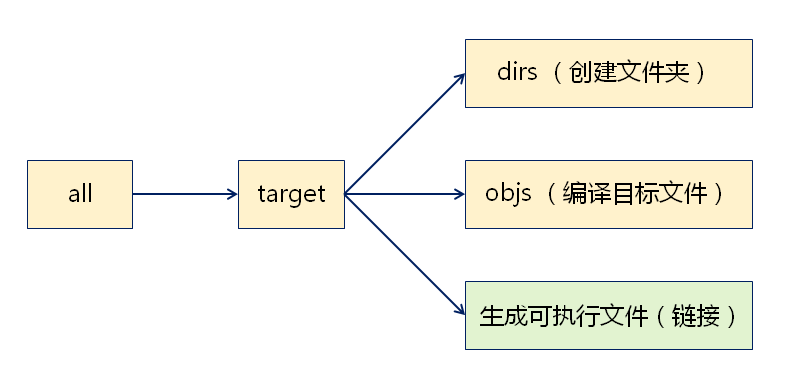5. 编程实现

CC := gcc
RM := rm -rf
MKDIR := mkdir

DIR_OBJS := objs
DIR_TARGETS := target

DIR := $(DIR_OBJS)$(DIR_TARGETS)

TARGET := $(DIR_TARGETS)/hello.out SRCS :=$(wildcard *.c)
OBJS := $(SRCS:.c=.o) OBJS :=$(addprefix $(DIR_OBJS)/,$(OBJS))

.PHONY := rebuild clean all

$(TARGET) :$(DIR) $(OBJS)$(CC) -o $@$(OBJS)
@echo "Target File ==> $@"$(DIR) :
$(MKDIR)$@

$(DIR_OBJS)/%.o : %.c ifeq ($(DEBUG),true)
$(CC) -o$@ -g -c $^ else$(CC) -o $@ -c$^
endif

clean :
$(RM)$(DIR)

rebuild : clean all

在当前目录下创建 objs target 文件，编译链接当前目录下 .c 文件，将生成的 .o 文件存放到 objs 文件夹，生成的可执行文件 app.out 存放在 target 文件夹，最后运行可执行程序 app.out 。其中当 DEBUG=true 时添加编译选项 -g 实现调试功能

十、include 关键字

1. include 关键字的处理方式

在当前目录搜索或指定目录搜索目标文件

1）搜索成功

类似于 c 语言中 include 关键字，将文件内容直接搬到 makefile 中, 编程实验如下

test.txt 文件中的内容

test :
@echo "this is test"

makefile 内容

.PHONY : all clean

include test.txt

all :
echo "this is all"

make all 运行结果：
this is test

2）搜索失败

产生警告，以文件作为目标查找并执行相应规则，当文件名对应的规则不存在时，最终产生错误

a）规则存在

test.txt 文件不存在

include test.txt

all :
@echo "this is all"

test.txt :
@echo "test.txt is not exist"

make all 运行结果：
makefile:2: test.txt: 没有那个文件或目录
test.txt is not exist
this is all

b）规则不存在

test.txt 文件不存在

include test.txt

all :
@echo "this is all"

make all 运行结果：
makefile:2: test.txt: 没有那个文件或目录
make: *** 没有规则可以创建目标“test.txt”。 停止。

2. include关键字使用总结

1）当目标文件不存在2）当目标文件存在3) 减号 - 的使用

使用减号 - 不但关闭了 include 发出的警告，同时关闭了错误；当错误发生时 make 将忽略这些错误

十一、自动生成依赖关系

1、编译行为带来的缺陷

预处理器直接将头文件中的代码直接插入源文件，编译器只通过预处理后的源文件产生目标文件，因此，规则中以源文件为依赖，修改头文件后，命令可能无法执行

2.、解决方案

可以将头文件作为依赖条件出现于每一个目标对应的规则中，当头文件改动，任何源文件都将被重新编译（编译低效），但是这种方案当项目中头文件数量巨大时，makefile 将很难维护

由于上述方案存在的缺陷，思考是否可以自动生成依赖解决,通过命令自动生成对头文件的依赖，将生成的依赖自动包含进 makefile 中，当头文件改动后，自动确认需要重新编译的文件。

3、预备知识

1) sed 流编辑器

sed 是一个流编辑器，用于流文本的修改（增/删/查/改），sed 可以用于流文本中的字符串替换，sed 字符串替换方式为：sed ‘s:src:des:g’。sed 字符串替换实例如下：

.PHONY : test

test :
@echo "test = > abc+abc=abc" | sed 's:abc:xyz:g'

test => xyz+xyz=xyz

sed 还支持正则表达式，在 sed 中可以用正则表达式匹配替换目标，并且可以使用匹配的目标生成替换结果

sed 's,$$.*$$\.o[ :]*,objs/\1.o : ,g'

gcc -M test.c

gcc -MM test.c

3) 拆分目标的依赖4) make 中的命令执行机制

.PHONY : all

all :
mkdir test
cd test
mkdir subtest
• 运行结果
期待的运行结果为，创建一个文件夹 test，在 test 中创建子文件夹 subtest，但实际运行结果为在当前目录下创建出两个文件夹分别为 test 和 subtest

• 运行结果分析
make 创建一个进程执行 mkdir test，执行完后进程结束，回到当前目录。make 又创建一个进程执行 cd test，执行完后进程结束，回到当前目录。make 再次创建一个进程执行 mkdir subtest，执行完后进程结束，回到当前目录。因此最终结果在当前目录下创建两个文件夹 test 和 subtest。

• 解决方案
set-e 指定发生错误后立即退出执行。可以通过分号 ; 和接续符 \ 将多个命令组合成一个命令。组合的命令一次在同一个进程中被执行，无论是否出错。

• 代码修改

.PHONY : all

all :
set -e; \
mkdir test; \
cd test; \
mkdir subtest

4、自动生成依赖关系

1）自动生成依赖文件

通过 include 指令包含所有的 .dep 依赖文件，当 .dep 依赖文件不存在时，使用规则自动生成

.PHONY : all clean

CC := gcc
RM := rm -rf
MKDIR := mkdir

SRCS := $(wildcard *.c) DEPS :=$(SRCS:.c=.dep)

-include $(DEPS) all : @echo all %.dep : %.c @echo "Creating$@..."
set -e;\
$(CC) -MM$^ | sed 's,$$.*$$\.o[ :]*,objs/\1.o : ,g' > $@ clean:$(RM) *.dep

Creating func.dep ...
Creating main.dep ...
make: Nothing to be done for 'objs/main.o'.

2) 集中管理 .dep 文件

当 include 发现 .dep 文件不存在，通过规则和命令创建 deps 文件夹将所有的 .dep 文件创建到 deps 文件夹.dep 文件中记录目标文件的依赖关系

.PHONY : all clean

MKDIR := mkdir
RM := rm -fr
CC := gcc

DIR_DEPS := deps

SRCS := $(wildcard *.c) DEPS :=$(SRCS:.c=.dep)
DEPS := $(addprefix$(DIR_DEPS)/,$(DEPS)) -include$(DEPS)

all :
@echo "all"

$(DIR_DEPS):$(MKDIR) $@$(DIR_DEPS)/%.dep : $(DIR_DEPS) %.c @echo "Creating$@ ..."
@set -e; \
$(CC) -MM -E$(filter %.c, $^) | sed 's,$$.*$$\.o[ :]*,objs/\1.o : ,g' >$@

clean :
$(RM)$(DIR_DEPS)

mkdir deps
Creating deps/sub.dep ...
Creating deps/a.dep ...
Creating deps/sub.dep ...
all

3) 一些 .dep 文件会被重复创建多次

deps 文件夹的时间属性会因为依赖文件创建而发生改变，make 发现 deps 文件夹比对应的目标更新，触发相应规则的重新解析和命令的执行

解决方案,添加宏代码块进行判断，当不存在 deps 文件添加文件依赖关系，否则取消文件依赖关系

ifeq ($(wildcard$(DIR_DEPS)), )
$(DIR_DEPS)/%.dep :$(DIR_DEPS) %.c
else
$(DIR_DEPS)/%.dep : %.c 4) 自动生成依赖的最终实现 .PHONY : all clean MKDIR := mkdir RM := rm -fr CC := gcc DIR_DEPS := deps DIR_OBJS := objs DIR_EXE := exes DIRS :=$(DIR_OBJS) $(DIR_EXE) APP =$(DIR_EXE)/app.out

SRCS := $(wildcard *.c) DEPS :=$(SRCS:.c=.dep)
DEPS := $(addprefix$(DIR_DEPS)/,$(DEPS)) OBJS :=$(SRCS:.c=.o)
OBJS := $(addprefix$(DIR_OBJS)/,$(OBJS)) all :$(APP)
./$(APP)$(APP) : $(DIRS)$(OBJS)
@echo "target ==> $@"$(CC) -o $@$(OBJS)

$(DIRS) :$(MKDIR) $@$(DIR_OBJS)/%.o : %.c
$(CC) -c$(filter %.c, $^) -o$@

ifeq ("$(MAKECMDGOALS)","all") -include$(DEPS)
endif

ifeq ("$(MAKECMDGOALS)","") -include$(DEPS)
endif

$(DIR_DEPS):$(MKDIR) $@ ifeq ($(wildcard $(DIR_DEPS)), )$(DIR_DEPS)/%.dep : $(DIR_DEPS) %.c else$(DIR_DEPS)/%.dep : %.c
endif
@echo "Creating $@ ..." @set -e; \$(CC) -MM -E $(filter %.c,$^) | sed 's,$$.*$$\.o[ :]*,objs/\1.o : ,g' > $@ clean :$(RM) $(DIR_DEPS)$(DIRS)

代码存在问题，当头文件中包含其他头文件时可能出现修改头文件，make 无法执行的情况。解决方案，将依赖文件名，作为目标加入自动生成的依赖关系中，通过 include 加载依赖文件时判断是否执行规则，在规则执行时重新生成依赖关系文件，最后加载新的依赖文件。即修改上述代码 52 行,在冒号前加入 $@$(CC) -MM -E $(filter %.c,$^) | sed 's,$$.*$$\.o[ :]*,objs/\1.o $@: ,g' >$@

十二、make 的隐式规则

1、makfile 中出现同名目标时

所有的依赖合并在一起，成为目标的最终依赖。当多处出现同一目标命令时，make 发出警告。所有之前定义的命令被最后定义的命令取代.

2、隐式规则

make 提供了一些常用的，预定义的规则实现。make 提供了生成目标文件的隐式规则，隐式规则会使用预定义变量完成编译工作，改变预定义变量将部分改变隐式规则的行为，当存在自定义规则时，不在使用隐式规则，当相应目标的规则未提供时，make 尝试使用隐式规则。

SRCS := $(wildcard *.c) OBJS :=$(SRCS:.c=.o)

app.out : $(OBJS)$(CC) -o $@$^
$(RM)$^
@echo "Target ==> $@" 运行结果: cc -c -o main.o main.c cc -c -o func.o func.c cc -o app.out main.o func.o rm -f main.o func.o Target ==> app.out 上述 makefile 没有$(OBJS) 的规则，为什么能正常运行，就是因为 make 的标准库中提供了对应的规则，当 make 发现目标的依赖不存在时，尝试通过依赖名逐一查找隐式规则，并且通过依赖名推到可能需要的源文件。最后根据文件后缀自动推导编译命令。

3、隐式规则的副作用

编译行为难以控制，大量使用隐式规则可能产生意想不到的编译行为。编译效率低下，make 从隐式规则和自定义规则中选择最终使用的规则。隐式规则链，当依赖目标存在时，make 会极力组合各种隐式规则对目标进行创建，进而产生意料之外的编译行为！查看隐式规则的方式如下。

make -p

make -p | grep "XXX"

除此之外还可以使用前面提到的 .VARIABLES 预定义变量查看 make 的自定义变量。我们可以通过下面方式禁用隐式规则。在 makefile 中自定义规则在 makefile 中自定义模式（如：%.o : %.c）全局禁用：make -r

4、后缀规则

后缀规则是旧式的“模式规则”，可以通过后缀描述的方式自定义规则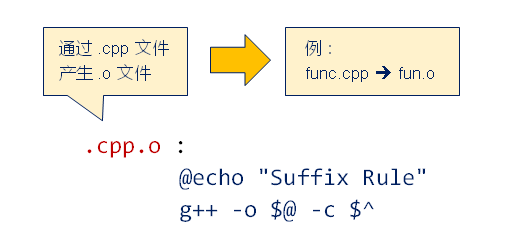双后缀规则，定义一对文件后缀（依赖文件后缀和目标文件后缀）

.cpp.o <==> %.o : %.cpp

单后缀规则，定义单个后缀文件（源文件后缀）

.c  <==> % : %.c

后缀规则需要注意，后缀规则中不允许有依赖。后缀规则必须有命令，否则无意义。后缀规则将逐步被模式规则取代。

十三、makefile 中的路径搜索

项目中的 makefile 必须能够正确的定位源文件和依赖文件，最终编译生成可执行程序。常用的源代码管理方式如下

Project
|
|
|--------->Module1
|             |
|             |--------->inc
|             |
|             |--------->src
|
|--------->Module2
|
|--------->inc
|
|--------->src

1、特殊的预定义变量 VPATH（全大写）

VPATH 变量的值用于指示 make 如何查找文件不同文件夹可作为 VPATH 的值同时出现文件夹的名字需要使用分隔符进行区分

VPATH := inc src （空格）
VPATH := inc;src （分号）
VPATH := inc:src （冒号）

1）make 对于 VAPATH 值得处理方式

当前文件夹找不到需要的文件时，VPATH会被使用，make 会在 VPATH 指定的文件夹中依次搜索文件，当多个文件夹存在同名文件时，选择第一次搜索到的文件

VPATH 只能决定 make 的搜索路径，无法决定命令的搜索路径

2）VAPATH 使用实例

.c 文件存放于 src 文件夹，.o 文件存放于 inc 文件夹，make 自动搜索相关路径生成可执行文件 app.out

.PHONY : all clean

MKDIR := mkdir
RM := rm -rf
CC := gcc

APP := app.out

DIR_INC := inc
DIR_SRC := src

VPATH := inc src

CFLAGS := -I$(DIR_INC) all :$(APP)
@echo "target ==> $(APP)"$(APP) : a.o sub.o add.o
$(CC) -o$@ $^ %.o : %.c$(CC) $(CFLAGS) -c$^ -o $@ clean :$(RM) *.o *.out

由于当多个文件夹存在同名文件时，选择第一次搜索到的文件这也会带来问题, 当 inc 文件意外出现源文件（c/cpp文件），那可能出现编译错误！可以使用 vpath 为不同类型的文件指定不同的搜索路径。

vpath Pattern Directory

在 Directory 下搜索符合 Pattern 的规则文件，将上述 VPATH 修改为 vpath

vpath %.h inc
vpath %.c src

2、取消搜索规则

取消已经设置的某个搜索规则

vpath Pattern

示例

vpath %.h inc  # 在 inc 中搜索 .h 文件
Vpath %h       # 不在 inc 中搜索 .h 文件

取消所有已经设置的规则

vpath

3、问题分析

• 当 VPATH 和 vpath 关键字同时出现，make 会如何处理？
make 首先在当前文件夹下搜索需要的文件，如果失败: make首先在 vpath 提供的路径下寻搜索目标文件。当搜索失败时，转而搜索 VPATH 指定的文件夹

• 当使用 vpath 对同一个 Pattern 指定多个文件夹时，make 会如何处理？
make 首先在当前文件夹搜索需要的文件，如果失败：make 以自上而下的顺序搜索 vpath 指定的文件夹。当找到目标文件，搜索结束

• 通过 VPATH 变量指定搜索路径后，make 如何决定目标文件的最终位置？
当 app.out 完全不存在，make 在当前文件夹下创建 app.out。当 src 文件中存在 app.out所有目标依赖的新旧关系不变，make 不会创建 app.out。当依赖文件被更新，make 在当前文件夹下创建 app.out

4、工程建议

尽量使用 vpath 为不同文件指定搜索路径、不要在源代码文件夹中生成目标文件、为编译得到的结果创立独立的文件夹、避免 VTPAH 和 GPATH 特殊变量的使用、只要使用 VPATH 就要考虑是否使用 GPATH

5、路径搜索的综合示例

1) 需求分析

• 工程项目中不希望源码文件夹在编译过程中被改动（只读文件夹）
• 在编译时自动创建文件夹（build）用于存放编译的结果
• 编译过程能够自动搜索需要的文件
• makefile 易于扩展，能够复用于相同类型的项目
• 支持调试版本的编译选项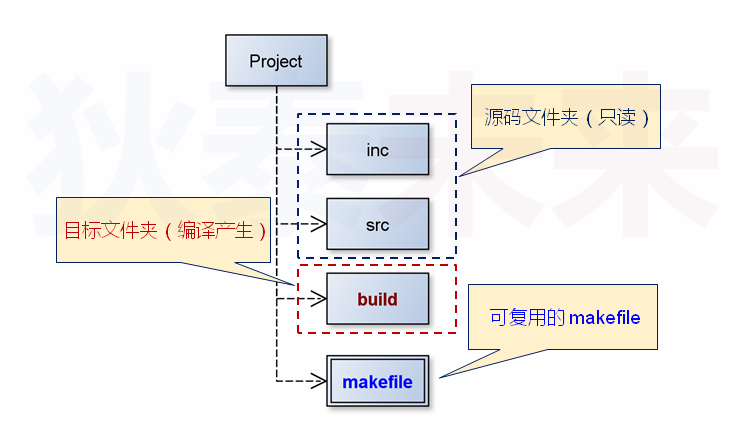OBJS := $(SRCS:.c=.o) 替换每一个目标的路径前缀（函数调用） OBJS :=$(patsubst src/%,build/%,$(OBJS)) 4) 编程实现 .PHONY : all clean MKDIR := mkdir RM := rm -rf CC := gcc DIR_INC := inc DIR_SRC := src DIR_BUILDS := build TYPE_INT := .h TYPE_SRC := .c TYPE_OBJ := .o DEBUG := LFLAGS := CFLAGS := -I$(DIR_INC)
ifeq ($(DEBUG),true) CFLAGS += -g endif APP :=$(DIR_BUILDS)/app.out

SCRS := $(wildcard$(DIR_SRC)/*$(TYPE_SRC)) OBJS :=$(SCRS:$(TYPE_SRC)=$(TYPE_OBJ))
OBJS := $(patsubst$(DIR_SRC)/%,$(DIR_BUILDS)/%,$(OBJS))
HDRS := $(wildcard$(DIR_INC)/*$(TYPE_INC)) HDRS :=$(notdir $(HDRS)) vpath %$(TYPE_INC) $(DIR_INC) vpath %$(TYPE_SRC) $(DIR_SRC) all :$(DIR_BUILDS) $(APP) ./$(APP)

$(DIR_BUILDS) :$(MKDIR) $@$(APP) : $(OBJS) @echo "target ==>$(APP)"
$(CC)$(LFLAGS) -o $@$^

$(DIR_BUILDS)/%$(TYPE_OBJ) : %$(TYPE_SRC)$(HDRS)
$(CC)$(CFLAGS) -c $< -o$@

clean :
$(RM)$(DIR_BUILDS)

十四、打造专业的编译环境

1、大型项目目录结构(无第三库)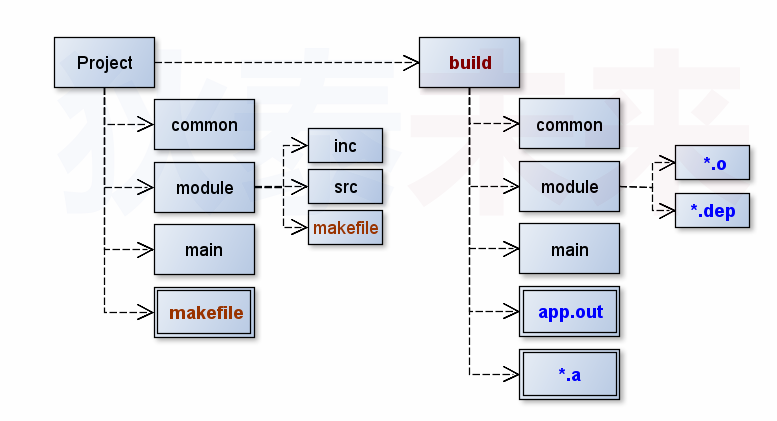2、需要打造的编译环境

• 源码文件夹在编译时不希望被改动（只读文件夹）
• 在编译时自动创建文件夹（build）用于存放编译结果
• 编译过程中自动生成依赖关系，自动搜索需要的文件
• 每个模块可以拥有自己独立的编译方式
• 支持调试版本的编译选项

3、项目架构设计分析

项目根据功能被划分为多个不同模块, 每个模块的代码用一个文件夹进行管理,文件夹由 inc, src, makfile 构成。每个模块的对外函数声明统一放置于 common/inc 中，如：common.h xxfunc.h

1）实现阶段分析

• Compile 阶段将每个文件中的代码编译成静态库文件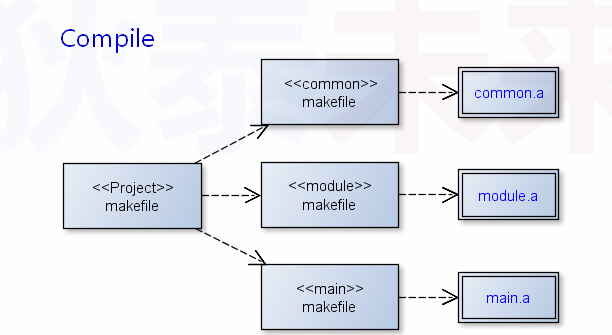因此 Compile 阶段，我们需要完成可用于各个模块可编译的 makefile 文件，每个模块的编译结果为静态库文件（.a文件）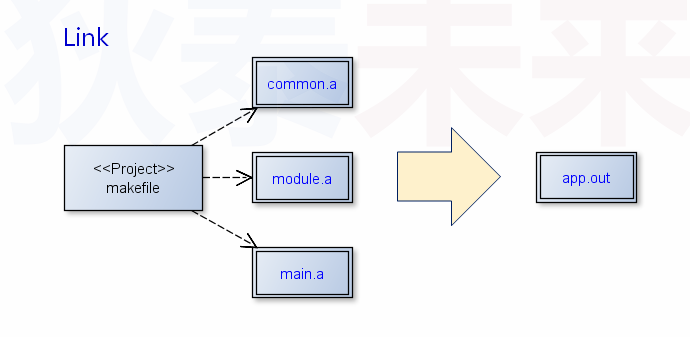因此 link 阶段，我们需要完成整编译个工程的 makefile 文件，调用模块的 makefile 编译生成静态库文件链接所有的静态库文件，最终得到可执行程序

a. Compile 阶段实现

关键的实现要点有三个，自动生成依赖关系（gcc -MM），自动搜索需要的文件（vpath），将目标打包为静态库文件（ar crs） 。模块中的 makefile 构成如下。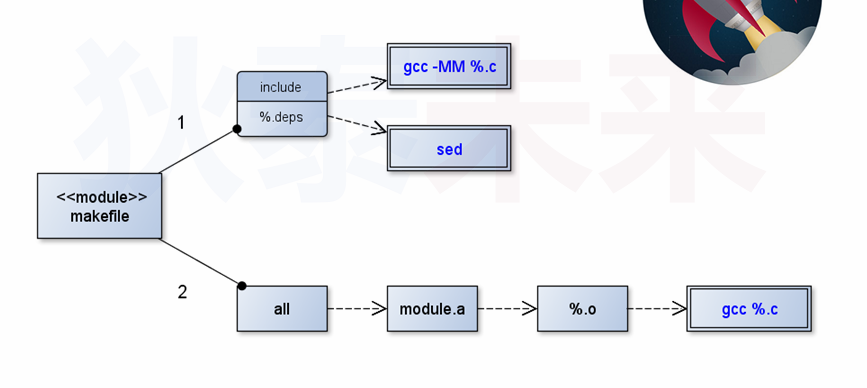编程实现

.PHONY : all

DIR_SRC := src
DIR_INC := inc
DIR_BUILD := /home/book/code/build
DIR_COMMON_INC := /home/book/code/common/inc

TYPE_INC := .h
TYPE_SRC := .c
TYPE_OBJ := .o
TYPE_DEP := .dep

AR := ar
ARFLAGS := crs

CC := gcc
CFLAGS := -I$(DIR_INC) -I$(DIR_COMMON_INC)

ifeq ($(DEBUG),true) CFLAGS += -g endif MODULE :=$(realpath .)       # 获取当前路径下的绝对路径
MODULE := $(notdir$(MODULE))

DIR_OUTPUT := $(addprefix$(DIR_BUILD)/,$(MODULE)) OUTPUT :=$(MODULE).a
OUTPUT := $(addprefix$(DIR_BUILD)/,$(OUTPUT)) SRCS :=$(wildcard $(DIR_SRC)/*$(TYPE_SRC))
OBJS := $(SRCS:$(TYPE_SRC)=$(TYPE_OBJ)) OBJS :=$(patsubst $(DIR_SRC)/%,$(DIR_OUTPUT)/%,$(OBJS)) DEPS :=$(SRCS:$(TYPE_SRC)=$(TYPE_DEP))
DEPS := $(patsubst$(DIR_SRC)/%, $(DIR_OUTPUT)/%,$(DEPS))

vpath %$(TYPE_INC)$(DIR_INC)
vpath %$(TYPE_INC)$(DIR_COMMON_INC)
vpath %$(TYPE_SRC)$(DIR_SRC)

include $(DEPS) all :$(OUTPUT)
@echo "Success! Target ==> $(OUTPUT)"$(OUTPUT) : $(OBJS)$(AR) $(ARFLAGS)$@ $^$(DIR_OUTPUT)/%$(TYPE_OBJ) : %$(TYPE_SRC)
$(CC)$(CFLAGS) -o $@ -c$(filter %$(TYPE_SRC),$^)

$(DIR_OUTPUT)/%$(TYPE_DEP) : %$(TYPE_SRC) @echo "Creating$@ ..."
@set -e; \
$(CC)$(CFLAGS) -MM -E $(filter %$(TYPE_SRC),$^) | sed 's,$$.*$$\.o[ :]*,$(DIR_OUTPUT)/\1.o $@: ,g' >$@

Link 阶段需要考虑的事情主要有四个，如何自动创建 build 文件夹以及子文件夹？如何进入每个模块进行编译？编译成功后如何链接所有的模块静态库？当前模块中有哪些模块？对于前三个问题比较简单分别是 使用 mkdir 创建 build 文件夹及子文件夹。使用 cd 命令进入模块编译。编译成功后使用 gcc 链接所有模块的静态库文件。需要解决的注意问题是第四个问题。

在实际的工程里面，项目中各个模块在设计阶段就已经基本确定，因此在在之后的开发过程中不会频繁的随意增加或者减少。因此可以直接定义变量模块名列表（模块变量名）利用 shell 的 for 循环遍历模块变量名在 for 循环中进入模块文件夹进行编译循环结束后链接所有的模块静态库文件

在 makefile 中插入 shell 的 for 循环的方式如下，需要注意的是 makefile 中嵌入 shell 代码时，如果需要使用 shell 变量的值，必须在变量前面加上 $$。 MODULES := common \ main \ module test : @for dir in (MODULES);\ do\ echo$$dir;\
done
@echo "Compile Success ..."

链接时需要注意，gcc 在进行静态库的链接时必须遵循严格的依赖关系

gcc -o app.out x.a y.a z.a

其中的依赖关系必须为：x.a --> y.a --> z.a ，默认情况下遵循自左向右的依赖关系。如果不清楚库间的依赖关系，可以使用 -Xlinker 自动确定依赖关系

MODULES := common \
main \
module

CC := gcc
MKDIR := mkdir
RM := rm -rf

LFLAGS :=

DIR_PROJECT := $(realpath .) DIR_BUILD := build DIR_BUILD_SUB :=$(addprefix $(DIR_BUILD)/,$(MODULES))
MODULE_LIB := $(addsuffix .a,$(MODULES))    # 增加后缀 .a
MODULE_LIB := $(addprefix$(DIR_BUILD)/,$(MODULE_LIB)) APP := app.out APP :=$(addprefix $(DIR_BUILD)/,$(APP))

link : $(MODULE_LIB) @echo "Bengin to link ..."$(CC) -o $(APP) -Xlinker "-("$^ -Xlinker "-)" $(LFLAGS) @echo "Success Target ==>$@ ..."

compile : $(DIR_BUILD)$(DIR_BUILD_SUB)
@echo "Compile Begin ..."
@set -e;\
for dir in $(MODULES);\ do\ cd $$dir && make all DEBUG:=(DEBUG) && cd ..;\ done @echo "Compile Success ..." (DIR_BUILD) (DIR_BUILD_SUB) : (MKDIR) @ clean : (RM) (DIR_BUILD) 2) 编译优化 上述 makefile 存在两个潜在问题，首先是所有模块 makefile 中使用编译路径均为写死的绝对路径，一旦项目文件夹移动，编译必将失败 针对这个问题在工程 makefile 中获取项目的源码目录，根据项目源码路径：拼接得到编译文件夹路径 DIR_BUILD，拼接得到全局头文件路径 DIR_COMMON_INC。通过定义命令行变量将路径传递给模块 makefile。优化后的代码如下： .PHONY : all compile link rebuild MODULES := common \ main \ module CC := gcc MKDIR := mkdir RM := rm -rf DIR_PROJECT := (realpath .) DIR_BUILD := build DIR_COMMON_INC := common/inc DIR_BUILD_SUB := (addprefix (DIR_BUILD)/,(MODULES)) MODULE_LIB := (addsuffix .a, (MODULES)) # 增加后缀 .a MODULE_LIB := (addprefix (DIR_BUILD)/,(MODULE_LIB)) APP := app.out APP := (addprefix (DIR_BUILD)/,(APP)) all : compile (APP) @echo "Target (APP) success!" compile : (DIR_BUILD) (DIR_BUILD_SUB) @echo "compile begin ..." @set -e;\ for dir in (MODULES); \ do \ cd$$dir && \$(MAKE) all \ # 传递路径给子 makefile
DEBUG:=$(DEBUG) \ DIR_BUILD:=$(addprefix $(DIR_PROJECT)/,$(DIR_BUILD)) \
DIR_COMMON_INC:=$(addprefix$(DIR_PROJECT)/,$(DIR_COMMON_INC)) && \ cd .. ;\ done @echo "compile success!"$(DIR_BUILD) $(DIR_BUILD_SUB) :$(MKDIR) $@ link$(APP) : $(MODULE_LIB) @echo "begin to link !"$(CC) -o $(APP) -Xlinker "-("$(MODULE_LIB) -Xlinker "-)"

clean :
$(RM)$(DIR_BUILD)

rebuild : clean all

第二个问题就是，所有 makefile 模块完全相同，当模块 makefile 需要改动时，将涉多处相同改动。针对这个问题，可以将模块 makefile 差分为两个模板文件 mod-cfg.mk 定义为可能改变的变量mod-rule.mk 定义相对稳定的变量和规则。默认情况下，模块 makefile 复用模板文件实现功能 (include)。这个解决方案需要，通过命令行变量进行模板文件位置的传递。优化后的 makefile 如下：

对于各个模块下的 makefile

include $(MOD_CFG) # Custmization Begin # # DIR_SRC := src # DIR_INC := inc # # TYPE_INC := .h # TYPE_SRC := .c # TYPE_OBJ := .o # TYPE_DEP := .dep # # Custmization End include$(CMD_CFG)

include $(MOD_RULE) 工程目录下的 makefile PHONY : all compile link rebuild MODULES := common \ main \ module CC := gcc MKDIR := mkdir RM := rm -rf DIR_PROJECT :=$(realpath .)
DIR_BUILD := build
DIR_COMMON_INC := common/inc
DIR_BUILD_SUB := $(addprefix$(DIR_BUILD)/,$(MODULES)) MODULE_LIB :=$(addsuffix .a, $(MODULES)) # 增加后缀 .a MODULE_LIB :=$(addprefix $(DIR_BUILD)/,$(MODULE_LIB))

MOD_CFG := mod-cfg.mk
MOD_RULE := mod-rule.mk
CMD_CFG := cmd-cfg.mk

APP := app.out
APP := $(addprefix$(DIR_BUILD)/,$(APP)) all : compile$(APP)
@echo "Target $(APP) success!" compile :$(DIR_BUILD) $(DIR_BUILD_SUB) @echo "compile begin ..." @set -e;\ for dir in$(MODULES); \
do \
cd $$dir && \ (MAKE) all \ DEBUG:=(DEBUG) \ DIR_BUILD:=(addprefix (DIR_PROJECT)/,(DIR_BUILD)) \ DIR_COMMON_INC:=(addprefix (DIR_PROJECT)/,(DIR_COMMON_INC)) \ MOD_CFG:=(addprefix (DIR_PROJECT)/,(MOD_CFG)) \ MOD_RULE:=(addprefix (DIR_PROJECT)/,(MOD_RULE))\ CMD_CFG:=(addprefix (DIR_PROJECT)/,(CMD_CFG))&& \ cd .. ;\ done @echo "compile success!" (DIR_BUILD) (DIR_BUILD_SUB) : (MKDIR) @ link (APP) : (MODULE_LIB) @echo "begin to link !" (CC) -o (APP) -Xlinker "-(" (MODULE_LIB) -Xlinker "-)" @echo "link success!" clean : (RM) (DIR_BUILD) rebuild : clean all 可能改变的变量 mod-cfg.mk DIR_SRC := src DIR_INC := inc TYPE_INC := .h TYPE_SRC := .c TYPE_OBJ := .o TYPE_DEP := .dep 定义相对稳定的变量和规则 mod-rule.mk .PHONY : all MODULE := (realpath .) # 获取当前路径下的绝对路径 MODULE := (notdir (MODULE)) DIR_OUTPUT := (addprefix (DIR_BUILD)/,(MODULE)) OUTPUT := (MODULE).a OUTPUT := (addprefix (DIR_BUILD)/,(OUTPUT)) SRCS := (wildcard (DIR_SRC)/*(TYPE_SRC)) OBJS := (SRCS:(TYPE_SRC)=(TYPE_OBJ)) OBJS := (patsubst (DIR_SRC)/%,(DIR_OUTPUT)/%,(OBJS)) DEPS := (SRCS:(TYPE_SRC)=(TYPE_DEP)) DEPS := (patsubst (DIR_SRC)/%, (DIR_OUTPUT)/%,(DEPS)) vpath %(TYPE_INC) (DIR_INC) vpath %(TYPE_INC) (DIR_COMMON_INC) vpath %(TYPE_SRC) (DIR_SRC) -include (DEPS) all : (OUTPUT) @echo "Success! Target ==> (OUTPUT)" (OUTPUT) : (OBJS) (AR) (ARFLAGS) @ ^ (DIR_OUTPUT)/%(TYPE_OBJ) : %(TYPE_SRC) (CC) (CFLAGS) -o @ -c (filter %(TYPE_SRC),^) (DIR_OUTPUT)/%(TYPE_DEP) : %(TYPE_SRC) @echo "Creating @ ..." @set -e; \ (CC) (CFLAGS) -MM -E (filter %(TYPE_SRC),^) | sed 's,$$.*$$\.o[ :]*,(DIR_OUTPUT)/\1.o @: ,g' > @ 定义相对稳定的命令 cmd-cfg.mk AR := ar ARFLAGS := crs CC := gcc LFLAGS := CFLAGS := -I(DIR_INC) -I(DIR_COMMON_INC) ifeq ((DEBUG),true) CFLAGS += -g endif 经过上述的拆分，已经解决了各个模块下的 makfile 复用的问题。但是对于工程 makefile 模块可以进一步进行优化。 可以拆分命令变量、项目变量、以及其他变量和规则到不同文件。 • cmd-cfg.mk ：定义命令相关变量 • pro-cfg.mk ：定义项目变量以及编译路径变量等 • pro-rule.mk ：定义其他变量和规则 • 最后的工程 makefile 通过包含拆分后的文件构成 (include) 优化后的 makeflie 如下, 首先是定义命令相关变量 cmd-cfg.mk AR := ar ARFLAGS := crs CC := gcc LFLAGS := CFLAGS := -I(DIR_INC) -I(DIR_COMMON_INC) ifeq ((DEBUG),true) CFLAGS += -g endif MKDIR := mkdir RM := rm -rf 定义项目变量以及编译路径变量等 pro-cfg.mk MODULES := common \ main \ module MOD_CFG := mod-cfg.mk MOD_RULE := mod-rule.mk CMD_CFG := cmd-cfg.mk DIR_BUILD := build DIR_COMMON_INC := common/inc APP := app.out 定义其他变量和规则 pro-rule.mk PHONY : all compile link rebuild DIR_PROJECT := (realpath .) DIR_BUILD_SUB := (addprefix (DIR_BUILD)/,(MODULES)) MODULE_LIB := (addsuffix .a, (MODULES)) # 增加后缀 .a MODULE_LIB := (addprefix (DIR_BUILD)/,(MODULE_LIB)) APP := (addprefix (DIR_BUILD)/,(APP)) all : compile (APP) @echo "Target (APP) success!" compile : (DIR_BUILD) (DIR_BUILD_SUB) @echo "compile begin ..." @set -e;\ for dir in (MODULES); \ do \ cd$$dir && \
$(MAKE) all \ DEBUG:=$(DEBUG) \
DIR_BUILD:=$(addprefix$(DIR_PROJECT)/,$(DIR_BUILD)) \ DIR_COMMON_INC:=$(addprefix $(DIR_PROJECT)/,$(DIR_COMMON_INC)) \
MOD_CFG:=$(addprefix$(DIR_PROJECT)/,$(MOD_CFG)) \ MOD_RULE:=$(addprefix $(DIR_PROJECT)/,$(MOD_RULE))\
CMD_CFG:=$(addprefix$(DIR_PROJECT)/,$(CMD_CFG))&& \ cd .. ;\ done @echo "compile success!"$(DIR_BUILD) $(DIR_BUILD_SUB) :$(MKDIR) $@ link$(APP) : $(MODULE_LIB) @echo "begin to link !"$(CC) -o $(APP) -Xlinker "-("$(MODULE_LIB) -Xlinker "-)"

clean :
$(RM)$(DIR_BUILD)

rebuild : clean all

最后工程 makefile 的内容就很简单了

include pro-cfg.mk   # 定义命令相关变量
include cmd-cfg.mk   # 定义项目变量以及编译路径变量等
include pro-rule.mk  # 定义其他变量和规则

到这里一个大型项目的编译环境就基本打造完成了。大型项目的编译环境是由不同的 makefile 构成的。编译环境的设计需要依据项目的整体架构设计。整个项目的编译可以分解为不同阶段。根据不同的阶段有针对性的对 makefile 进行设计。makefile 也需要考虑复用性和维护性等基本程序特性。

11-051102-06120
02-082078
02-0692
02-06134
02-08228
12-20506
08-2361
02-06770
02-06542
02-06174
08-23120
02-23619
02-08173
02-24154
02-11255
02-09226
02-11172
02-23516baron-z

¥2 ¥4 ¥6 ¥10 ¥20余额支付 (余额：-- )扫码支付获取中扫码支付点击重新获取扫码支付1.余额是钱包充值的虚拟货币，按照1:1的比例进行支付金额的抵扣。
2.余额无法直接购买下载，可以购买VIP、C币套餐、付费专栏及课程。余额充值